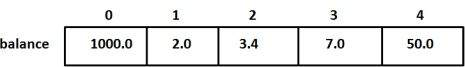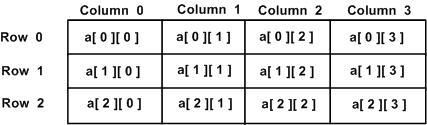# D Programming - Arrays

D programming language provides a data structure, named arrays, which stores a fixed-size sequential collection of elements of the same type. An array is used to store a collection of data. It is often more useful to think of an array as a collection of variables of the same type.

Instead of declaring individual variables, such as number0, number1, ..., and number99, you declare one array variable such as numbers and use numbers, numbers, and ..., numbers to represent individual variables. A specific element in an array is accessed by an index.

All arrays consist of contiguous memory locations. The lowest address corresponds to the first element and the highest address to the last element.

## Declaring Arrays

To declare an array in D programming language, the programmer specifies the type of the elements and the number of elements required by an array as follows −

```type arrayName [ arraySize ];
```

This is called a single-dimension array. The arraySize must be an integer constant greater than zero and type can be any valid D programming language data type. For example, to declare a 10-element array called balance of type double, use this statement −

```double balance;
```

## Initializing Arrays

You can initialize D programming language array elements either one by one or using a single statement as follows

```double balance = [1000.0, 2.0, 3.4, 17.0, 50.0];
```

The number of values between square brackets[ ] on right side cannot be larger than the number of elements you declare for the array between square brackets [ ]. The following example assigns a single element of the array −

If you omit the size of the array, an array just big enough to hold the initialization is created. Therefore, if you write

```double balance[] = [1000.0, 2.0, 3.4, 17.0, 50.0];
```

then you will create exactly the same array as you did in the previous example.

```balance = 50.0;
```

The above statement assigns element number 5th in the array a value of 50.0. Array with 4th index will be 5th, i.e., last element because all arrays have 0 as the index of their first element which is also called base index. The following pictorial representaion shows the same array we discussed above −## Accessing Array Elements

An element is accessed by indexing the array name. This is done by placing the index of the element within square brackets after the name of the array. For example −

```double salary = balance;
```

The above statement takes 10th element from the array and assigns the value to the variable salary. The following example implements declaration, assignment, and accessing arrays −

```import std.stdio;
void main() {
int n[ 10 ]; // n is an array of 10 integers

// initialize elements of array n to 0
for ( int i = 0; i < 10; i++ ) {
n[ i ] = i + 100; // set element at location i to i + 100
}

writeln("Element \t Value");

// output each array element's value
for ( int j = 0; j < 10; j++ ) {
writeln(j," \t ",n[j]);
}
}
```

When the above code is compiled and executed, it produces the following result −

```Element   Value
0         100
1         101
2         102
3         103
4         104
5         105
6         106
7         107
8         108
9         109
```

## Static Arrays Versus Dynamic Arrays

If the length of an array is specified while writing program, that array is a static array. When the length can change during the execution of the program, that array is a dynamic array.

Defining dynamic arrays is simpler than defining fixed-length arrays because omitting the length makes a dynamic array −

```int[] dynamicArray;
```

## Array Properties

Here are the properties of arrays −

Sr.No. Property & Description
1

.init

Static array returns an array literal with each element of the literal being the .init property of the array element type.

2

.sizeof

Static array returns the array length multiplied by the number of bytes per array element while dynamic arrays returns the size of the dynamic array reference, which is 8 in 32-bit builds and 16 on 64-bit builds.

3

.length

Static array returns the number of elements in the array while dynamic arrays is used to get/set number of elements in the array. Length is of type size_t.

4

.ptr

Returns a pointer to the first element of the array.

5

.dup

Create a dynamic array of the same size and copy the contents of the array into it.

6

.idup

Create a dynamic array of the same size and copy the contents of the array into it. The copy is typed as being immutable.

7

.reverse

Reverses in place the order of the elements in the array. Returns the array.

8

.sort

Sorts in place the order of the elements in the array. Returns the array.

### Example

The following example explains the various properties of an array −

```import std.stdio;

void main() {
int n[ 5 ]; // n is an array of 5 integers

// initialize elements of array n to 0
for ( int i = 0; i < 5; i++ ) {
n[ i ] = i + 100; // set element at location i to i + 100
}

writeln("Initialized value:",n.init);

writeln("Length: ",n.length);
writeln("Size of: ",n.sizeof);
writeln("Pointer:",n.ptr);

writeln("Duplicate Array: ",n.dup);
writeln("iDuplicate Array: ",n.idup);

n = n.reverse.dup;
writeln("Reversed Array: ",n);

writeln("Sorted Array: ",n.sort);
}
```

When the above code is compiled and executed, it produces the following result −

```Initialized value:[0, 0, 0, 0, 0]

Length: 5
Size of: 20

Pointer:7FFF5A373920
Duplicate Array: [100, 101, 102, 103, 104]
iDuplicate Array: [100, 101, 102, 103, 104]
Reversed Array: [104, 103, 102, 101, 100]
Sorted Array: [100, 101, 102, 103, 104]
```

## Multi Dimensional Arrays in D

D programming allows multidimensional arrays. Here is the general form of a multidimensional array declaration −

```type name[size1][size2]...[sizeN];
```

### Example

The following declaration creates a three dimensional 5 . 10 . 4 integer array −

```int threedim;
```

## Two-Dimensional Arrays in D

The simplest form of the multidimensional array is the two-dimensional array. A two-dimensional array is, in essence, a list of one-dimensional arrays. To declare a two-dimensional integer array of size [x, y] you would write syntax as follows −

```type arrayName [ x ][ y ];
```

Where type can be any valid D programming data type and arrayName will be a valid D programming identifier.

Where type can be any valid D programming data type and arrayName is a valid D programming identifier.

A two-dimensional array can be thought as a table, which has x number of rows and y number of columns. A two-dimensional array a containing three rows and four columns can be shown as below −Thus, every element in array a is identified by an element as a[ i ][ j ], where a is the name of the array, and i and j are the subscripts that uniquely identify each element in a.

## Initializing Two-Dimensional Arrays

Multidimensioned arrays may be initialized by specifying bracketed values for each row. The following array has 3 rows and each row has 4 columns.

```int a = [
[0, 1, 2, 3] ,   /*  initializers for row indexed by 0 */
[4, 5, 6, 7] ,   /*  initializers for row indexed by 1 */
[8, 9, 10, 11]   /*  initializers for row indexed by 2 */
];
```

The nested braces, which indicate the intended row, are optional. The following initialization is equivalent to previous example −

```int a = [0,1,2,3,4,5,6,7,8,9,10,11];
```

## Accessing Two-Dimensional Array Elements

An element in 2-dimensional array is accessed using the subscripts, means row index and column index of the array. For example

```int val = a;
```

The above statement takes 4th element from the 3rd row of the array. You can verify it in the above digram.

```import std.stdio;

void main () {
// an array with 5 rows and 2 columns.
int a = [ [0,0], [1,2], [2,4], [3,6],[4,8]];

// output each array element's value
for ( int i = 0; i < 5; i++ ) for ( int j = 0; j < 2; j++ ) {
writeln( "a[" , i , "][" , j , "]: ",a[i][j]);
}
}
```

When the above code is compiled and executed, it produces the following result −

```a: 0
a: 0
a: 1
a: 2
a: 2
a: 4
a: 3
a: 6
a: 4
a: 8
```

## Common Array Operations in D

Here are various operations performed on the arrays −

### Array Slicing

We often use part of an array and slicing array is often quite helpful. A simple example for array slicing is shown below.

```import std.stdio;

void main () {
// an array with 5 elements.
double a = [1000.0, 2.0, 3.4, 17.0, 50.0];
double[] b;

b = a[1..3];
writeln(b);
}
```

When the above code is compiled and executed, it produces the following result −

```[2, 3.4]
```

### Array Copying

We also use copying array . A simple example for array copying is shown below.

```import std.stdio;

void main () {
// an array with 5 elements.
double a = [1000.0, 2.0, 3.4, 17.0, 50.0];
double b;
writeln("Array a:",a);
writeln("Array b:",b);

b[] = a;      // the 5 elements of a are copied into b
writeln("Array b:",b);

b[] = a[];   // the 5 elements of a are copied into b
writeln("Array b:",b);

b[1..2] = a[0..1]; // same as b = a
writeln("Array b:",b);

b[0..2] = a[1..3]; // same as b = a, b = a
writeln("Array b:",b);
}
```

When the above code is compiled and executed, it produces the following result −

```Array a:[1000, 2, 3.4, 17, 50]
Array b:[nan, nan, nan, nan, nan]
Array b:[1000, 2, 3.4, 17, 50]
Array b:[1000, 2, 3.4, 17, 50]
Array b:[1000, 1000, 3.4, 17, 50]
Array b:[2, 3.4, 3.4, 17, 50]
```

### Array Setting

A simple example for setting value in an array is shown below.

```import std.stdio;

void main () {
// an array with 5 elements.
double a;
a[] = 5;
writeln("Array a:",a);
}
```

When the above code is compiled and executed, it produces the following result −

```Array a:[5, 5, 5, 5, 5]
```

### Array Concatenation

A simple example for concatenation of two arrays is shown below.

```import std.stdio;

void main () {
// an array with 5 elements.
double a = 5;
double b = 10;
double [] c;
c = a~b;
writeln("Array c: ",c);
}
```

When the above code is compiled and executed, it produces the following result −

```Array c: [5, 5, 5, 5, 5, 10, 10, 10, 10, 10]
```